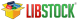`IIR Filter Library  mikroC PRO for PIC32 Libraries > DSP Libraries > ` `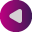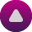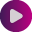`

## IIR Filter Library

mikroC PRO for PIC32 includes a library for Infinite Impulse Response (IIR) filter. All routines work with fractional Q15 format.

A infinite impulse response (IIR) filter is a type of a digital filter, whose impulse response (the filter's response to a delta function) is non-zero over an infinite length of time.

### IIR_Radix

Prototype int IIR_Radix (const int BScale, const int AScale, const int *ptrB, const int *ptrA, unsigned FilterOrder, int *ptrInput, unsigned InputLength, int *ptrOutput, unsigned Index); This function applies IIR filter to `ptrInput`. `BScale:` B scale factor. `AScale:` A scale factor. `ptrB:` pointer to B coefficients (in program memory). `ptrA:` pointer to A coefficients (in program memory). `FilterOrder:` order of the filter + 1. `ptrInput:` address of input samples. `InputLen:` number of samples. `ptrOutput:` pointer to output samples. Output length is equal to Input length. `Index:` index of current sample. Function returns value of the `Index`-th sample of the filtered signal :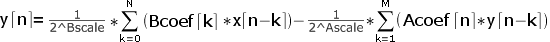Nothing. ```// Filter setup: // Filter kind: IIR // Filter type: Lowpass filter // Filter order: 2 // Design method: Butterworth const unsigned int FILTER_ORDER = 2; const unsigned int COEFF_B[FILTER_ORDER+1] = {0x344D, 0x689A, 0x344D}; const unsigned int COEFF_A[FILTER_ORDER+1] = {0x4000, 0xA003, 0x2687}; const unsigned int SCALE_B = 4; const unsigned int SCALE_A = -1; int inputSamples = {0x4000, 0x2000, 0x1000}; int outputSamples; int i; void main() { for(i = 0; i < 7; i++) outputSamples[i] = IIR_Radix(SCALE_B, SCALE_A, COEFF_B, COEFF_A, FILTER_ORDER+1, inputSamples, 3,outputSamples, i); // outputSamples = 1/(2^SCALE_B)*COEFF_B*inputSamples // outputSamples = 1/(2^SCALE_B) * (COEFF_B*inputSamples + COEFF_B*inputSamples) - 1/(2^SCALE_A)*(COEFF_A*outputSamples) // . // . // . } ``` None.
Copyright (c) 2002-2012 mikroElektronika. All rights reserved.
What do you think about this topic ? Send us feedback!
Want more examples and libraries?
Find them on